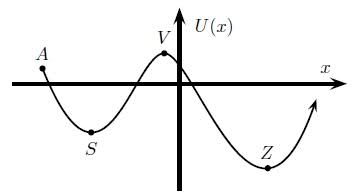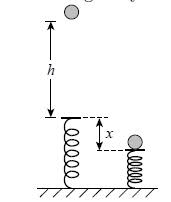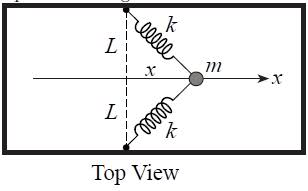# A few questions, conservation of energy

PinguNinjitsu

## Homework Statement

1.)A particle is released from point A and
moves in the potential U(x). Suppose the
mechanical energy of the system is conserved.
At which position(s) will the kinetic energy
of the particle have its maximum value?2.)A(n) 126 g ball is dropped from a height of
58.1 cm above a spring of negligible mass.
The ball compresses the spring to a maximum
displacement of 4.85169 cm.
The acceleration of gravity is 9.8 m/s2 .
Calculate the spring force constant k.
Answer in units of N/m.3.)A 4 kg block moving along the x axis is acted
upon by a single horizontal force that varies
with the block’s position according to the equation
Fx = a x2 + b ,
where a = 6 N/m2, and b = −4 N. At 1.6 m,
the block is moving to the right with a speed
of 4.6 m/s.
Determine the speed of the block at 2.2 m.
Answer in units of m/s.

4.)The potential energy between two atoms in a
particular molecule has the form
U(x) =(2.6)/x^8 −(5.1)/x^4
where the units of x are length and the num-
bers 2.6 and 5.1 have appropriate units so
that U(x) has units of energy.
What is the equilibrium separation of the
atoms (that is the distance at which the force
between the atoms is zero)?

5.)A particle of mass 8.95 kg is attached to two
identical springs on a horizontal frictionless
tabletop as shown.
The springs have spring constant 37.7 N/m
and equilibrium length L = 0.74 m.
If the mass is pulled 0.428 m to the right
and then released, what is its speed when it
reaches the equilibrium point x = 0?
Answer in units of m/s.## Homework Equations

k=.5mv^2
u=mgh
W=FD
u(spring)=1/2kx^2
F=-du/dx

## The Attempt at a Solution

1.) I guess I don't really understand what to look for in this graph, I know that for a negative slope the force is positive and S and Z are neutral equilibriums and V is an unstable equilibrium. I was not sure how to determine the maxiumu KE though..
2.) I got k=609.56 N/m for this problem but it was wrong, and I am not sure why. This is what I did:
Ui(ball)+Ui(spring)=Uf(ball)+Uf(spring)=mgh+.5kx^2=mgh+.5kx^2=(.126*9.8*.581)=.5k(.0485169)^2
And then I got k=609.56N/m But this was wrong, and I'm not sure what I was doing wrong.
3.) I got v=5.393978124 but Im unsure if I worked this out correctly
First I took the first d=1.6m and v=4.6m/s and plugged it into W=FD=KE=1/2mv^2 and got x as 2.252776065 then I took the second value of d=2.2 and used it in the same equation as be fore to get v. Now I am really not sure if this works at all, I just didn't know how to go about it.
4.) for this problem I took F=0 because there would not be force if the atoms were at equilibrium, I got the equation for F from f=-du/dx and then from there I solved for x and go 1.004866324. Now I'm not sure if that is at all right, and I don't know if that is the distance I am looking for.
5.) I honestly just don't know what to do for this one >.<

Help on any of these problems would be greatly appreciated I got to all the lectures and read the book, but unfortunately I seem to be failing when it comes to applying things. I know these are a lot of questions but help would be appreciated!

Last edited:

## Answers and Replies

xcvxcvvc
for #1, if U(x) is potential energy and all energy is conserved, what happens if potential energy decreases? What place can it only transfer, i.e. what increases when potential energy decreases?

for #2, you forgot the gravitational potential energy released when it went below height h and into compression x. You can account for it by either adding to the h on the left side or by subtracting mgx on the right side.

for #3, I'd integrate from 1.6 to 2.2 to find the work done by the force and then say final kinetic energy = work done by force + initial kinetic energy